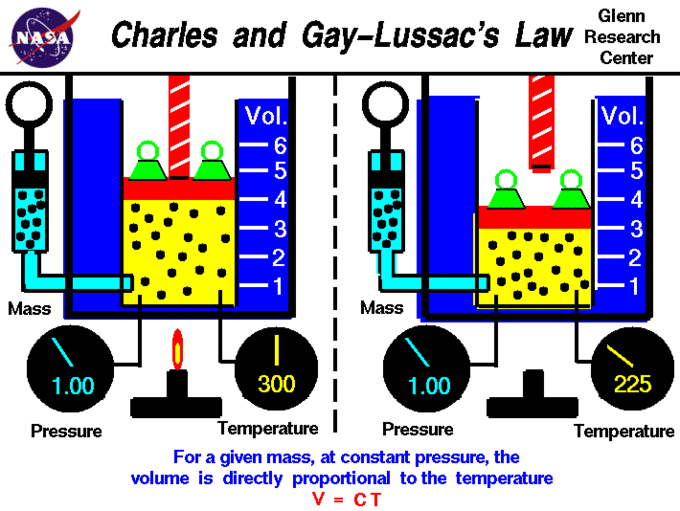## Charles’ and Gay-Lussac’s Law: Temperature and Volume

#### Learning Objective

• State Charles’ Law and its underlying assumptions

#### Key Points

• The lower the pressure of a gas, the greater its volume (Boyle’s Law); at low pressures, $\frac{V}{273}$ will have a larger value.
• Charles’ and Gay-Lussac’s Law can be expressed algebraically as $\frac{\Delta V}{\Delta T} = constant$ or $\frac{V_1}{T_1} = \frac{V_2}{T_2}$ .

#### Terms

• absolute zerothe theoretical lowest possible temperature; by international agreement, absolute zero is defined as 0 K on the Kelvin scale and as −273.15° on the Celsius scale
• Charles’ lawat constant pressure, the volume of a given mass of an ideal gas increases or decreases by the same factor as its temperature on the absolute temperature scale (i.e. gas expands as temperature increases)

## Charles’ and Guy-Lussac’s Law

Charles’ Law describes the relationship between the volume and temperature of a gas. The law was ﬁrst published by Joseph Louis Gay-Lussac in 1802, but he referenced unpublished work by Jacques Charles from around 1787. This law states that at constant pressure, the volume of a given mass of an ideal gas increases or decreases by the same factor as its temperature (in Kelvin); in other words, temperature and volume are directly proportional. Stated mathematically, this relationship is:

$\frac {V_1}{T_1}=\frac{V_2}{T_2}$

## Example

• A car tire filled with air has a volume of 100 L at 10°C. What will the expanded volume of the tire be after driving the car has raised the temperature of the tire to 40°C?
• $\frac {V_1}{T_1}=\frac{V_2}{T_2}$
• $\frac {\text{100 L}}{\text{283 K}}=\frac{V_2}{\text{313 K}}$
• $V_2=\text{110 L}$

## V vs. T Plot and Charles’ Law

A visual expression of Charles’ and Gay-Lussac’s Law is shown in a graph of the volume of one mole of an ideal gas as a function of its temperature at various constant pressures. The plots show that the ratio $\frac{V}{T}$ (and thus $\frac{\Delta V}{\Delta T}$) is a constant at any given pressure. Therefore, the law can be expressed algebraically as $\frac{\Delta V}{\Delta T} = \text{constant}$ or $\frac{V_1}{T_1} = \frac{V_2}{T_2}$.Charles’ and Gay–Lussac’s LawA visual expression of the law of Charles and Gay-Lussac; specifically, a chart of the volume of one mole of an ideal gas as a function of its temperature at various constant pressures.

## Extrapolation to Zero Volume

If a gas contracts by 1/273 of its volume for each degree of cooling, it should contract to zero volume at a temperature of –273°C; this is the lowest possible temperature in the universe, known as absolute zero. This extrapolation of Charles’ Law was the first evidence of the significance of this temperature.

## Why Do the Plots for Different Pressures Have Different Slopes?

The lower a gas’ pressure, the greater its volume (Boyle’s Law), so at low pressures, the fraction \frac{V}{273} will have a larger value; therefore, the gas must “contract faster” to reach zero volume when its starting volume is larger.